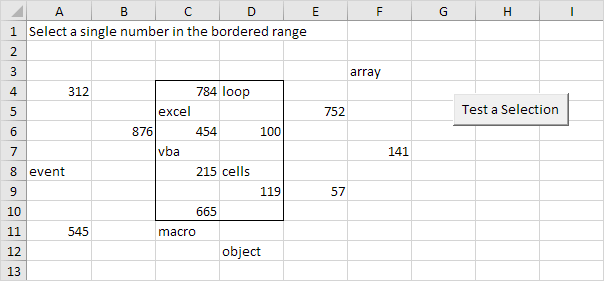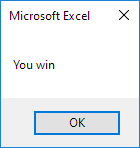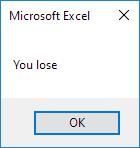# Test a Selection

This program in Excel VBA uses the Count property, IsNumeric function, IsEmpty function and Intersect method to test a selection.

Situation:Place a command button on your worksheet and add the following code lines:

1. First, we declare two Range objects. We call the Range objects rng and cell.

Dim rng As Range, cell As Range

2. We initialize the Range object rng with Range("C4:D10") and the Range object cell with the selected range.

Set rng = Range("C4:D10")
Set cell = Selection

3. Add the following code lines:

If cell.Count = 1 And IsNumeric(cell) And Not IsEmpty(cell) And Not Intersect(rng, cell) Is Nothing Then
MsgBox "You win"
Else
MsgBox "You lose"
End If

Explanation: Intersect(rng, cell) returns a Range object that represents the intersection of the two ranges. If this range object is not Nothing, the single number (first three conditions) is in the bordered range.

4. Test the program.

Only when you select a single number in the bordered range the result will be:In all other cases the result will be:Go to Next Chapter: Variables